# Algebra II : Mean

## Example Questions

← Previous 1 3 4 5 6 7 8 9 10 11

### Example Question #1 : Mean

The mean of the following numbers is 12. Solve for x.

14, 11, 10, 8, x

Possible Answers: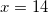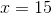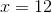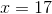Correct answer:Explanation: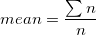where n equals the number of events.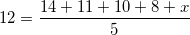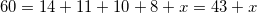### Example Question #29 : How To Find F(X)

There exists a function f(x) = 3x + 2 for x = 2, 3, 4, 5, and 6. What is the average value of the function?

Possible Answers:

20

14

25

4

6

Correct answer:

14

Explanation:

First we need to find the values of the function: f(2) = 3 * 2 + 2 = 8, f(3) = 11, f(4) = 14, f(5) = 17, and f(6) = 20. Then we can take the average of the five numbers:

average = (8 + 11 + 14 + 17 + 20) / 5 = 14

### Example Question #2 : Mean

On four exams, John has an average score of 94 points. His lowest score was an 89. If this score is dropped, what is his new average?

Possible Answers: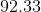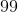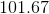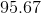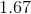Correct answer:Explanation:

To answer this question, you must first calculate John's total points from the four exams. The total points will be equal to four times the original average: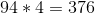Now, if the 89 is removed, his new total will be: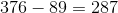With the score dropped, his new total number of exams is 3. Therefore, the new average is: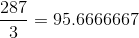Round this up to 95.67.

### Example Question #3 : Mean

After five exams, Isidore has an average score of 88 points. He then takes two more exams, earning a 77 and a 103. What is his new average?

Possible Answers: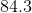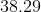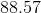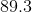Correct answer:Explanation:

To solve this, first calculate the total number of points that Isidore has earned. To find this total, multiply his original average by the original number of exams: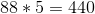Then, to this you must add his new scores to get the new total: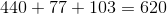After taking the two new exams, he has 7 total exams. Therefore, his average is: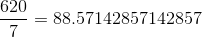This rounds to an 88.57.

### Example Question #4 : Mean

Sally is five inches taller than Mike, who is three inches shorter than Patrick. Patrick is 67 inches tall. What is the mean height of the three people?

Possible Answers: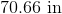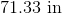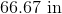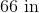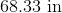Correct answer:Explanation:

To calculate the mean, you will need to figure out each person's height. Start with what we know.

Patrick is 67 inches tall: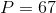.

Mike is three inches shorter than Patrick: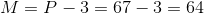.

Sally is five inches taller than Mike: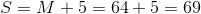.

Find the sum of their heights: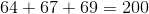Their average height is therefore: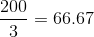### Example Question #5 : Mean

In a bed of plants, six are 55 inches tall, twelve are 70 inches tall, and three are 80 inches tall. To the nearest hundreth, what is the average height of the plants?

Possible Answers: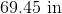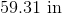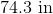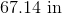Correct answer:Explanation:

To answer this, you need to know the total inches of all the plants. You must multiply the number of each type by its respective height: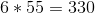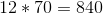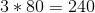Then, add these together: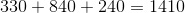Now, the total number of plants is: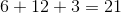The mean (or average) of this group is: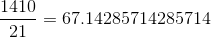Round this to the nearest hundreth to get 67.14 inches.

Peter was paid $4000 for editing a 3200-page encyclopedia. What was his rate earned per page? Possible Answers: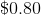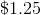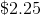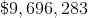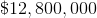Correct answer:Explanation: This question is really just asking for the average dollar per page. This could be easily calculated:However, you might also think of this as a rate problem: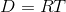Using the information from the question, we can see that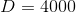and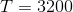. This comes out to what we solved above: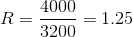### Example Question #7 : Mean A group of five candles are burning. Each candle is 1.5 inches taller than the one to its left. The smallest candle is 5 inches tall. What is the mean height of the group? Possible Answers: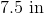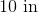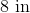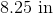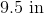Correct answer:Explanation: To solve this, you should first calculate the size of the candles. Based on the description, the group would be:The sum of this group is: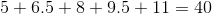The average is then found by dividing this total by 5: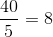### Example Question #8 : Mean A bank has 100 accounts established. The smallest account contains$1000. Each account after this one is $100 more than the one previous. For instance, the next account is$1100, then the next $1200, and so forth. What is the mean value of the accounts in the bank? Possible Answers: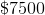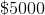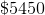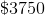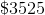Correct answer:Explanation: The easiest way to think of this problem is to make a list of the accounts (though not all 100, of course). The largest account will be: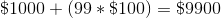. So, our list looks like this: 1000, 1100, 1200, . . ., 9700, 9800, 9900 Now we need to identify a pattern. We can pair the first and the last element: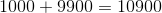Now, notice what happens when we pair the second and the second from the last element: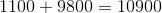This pattern is going to work for the whole list! If there are 100 total accounts, that means that we can make 50 such pairs. That means that we have 50 pairs of$10900. The total account amount is therefore: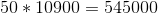The mean will be equal to this total value divided by the number of accounts (100):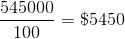### Example Question #9 : Mean

Find the mean of the following data set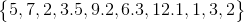Possible Answers: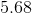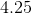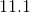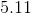Correct answer:Explanation:

The mean is the average of a set of data. In order to calculate this value, we need to take the sum of all the numbers and divide that by the number of values we have: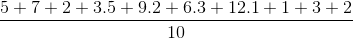This gives us: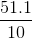which finally gives us a mean of:← Previous 1 3 4 5 6 7 8 9 10 11

### All Algebra II Resources